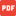International1m x 1m.pdf1m x 2m, long axis.pdf1m x 2m, short axis.pdf1m x 3m, long axis.pdf1m x 3m, short axis.pdf1m x 4m, long axis.pdf1m x 4m, short axis.pdf1m x 5m, long axis.pdf1m x 5m, short axis.pdf1m x 6m, long axis.pdf1m x 6m, short axis.pdf1m x 7m, long axis.pdf1m x 7m, short axis.pdf2m x 2m.pdf2m x 3m, long axis.pdf2m x 3m, short axis.pdf2m x 4m, long axis.pdf2m x 4m, short axis.pdf2m x 5m, long axis.pdf2m x 5m, short axis.pdf2m x 6m, long axis.pdf2m x 6m, short axis.pdf2m x 7m, long axis.pdf2m x 7m, short axis.pdf3m x 3m.pdf3m x 4m, long axis.pdf3m x 4m, short axis.pdf3m x 5m, long axis.pdf3m x 5m, short axis.pdf3m x 6m, long axis.pdf3m x 6m, short axis.pdf3m x 7m, long axis.pdf3m x 7m, short axis.pdf4m x 4m.pdf4m x 5m, long axis.pdf4m x 5m, short axis.pdf4m x 6m, long axis.pdf4m x 6m, short axis.pdf4m x 7m, long axis.pdf4m x 7m, short axis.pdf5m x 5m.pdf5m x 6m, long axis.pdf5m x 6m, short axis.pdf5m x 7m, long axis.pdf5m x 7m, short axis.pdf6m x 6m.pdf6m x 7m, long axis.pdf6m x 7m, short axis.pdf7m x 7m.pdf